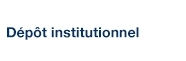# Words and Polynomial Invariants of Finite Groups in Non-Commutative Variables

Bergeron-Brlek, Anouk; Hohlweg, Christophe et Zabrocki, Mike (2011). « Words and Polynomial Invariants of Finite Groups in Non-Commutative Variables ». Annals of Combinatorics, 16(1), pp. 1-36.

Fichier(s) associé(s) à ce document :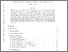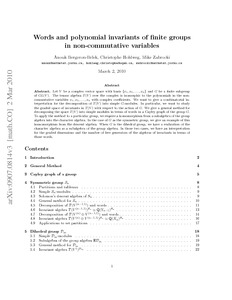Prévisualisation
PDF
Télécharger (485kB)

## Résumé

Let V be a complex vector space with basis {x 1, x 2, . . . , x n } and G be a finite subgroup of GL(V). The tensor algebra T(V) over the complex is isomorphic to the polynomials in the non-commutative variables x 1, x 2, . . . , x n with complex coefficients. We want to give a combinatorial interpretation for the decomposition of T(V) into simple G-modules. In particular, we want to study the graded space of invariants in T(V) with respect to the action of G. We give a general method for decomposing the space T(V) into simple modules in terms of words in a Cayley graph of the group G. To apply the method to a particular group, we require a homomorphism from a subalgebra of the group algebra into the character algebra. In the case of G as the symmetric group, we give an example of this homomorphism from the descent algebra. When G is the dihedral group, we have a realization of the character algebra as a subalgebra of the group algebra. In those two cases, we have an interpretation for the graded dimensions and the number of free generators of the algebras of invariants in terms of those words.

Type: Article de revue scientifique non-commutative invariant theory, representation theory, finite groups, symmetric group, dihedral group, Cayley graphs, words Faculté des sciences > Département de mathématiques Christophe Hohlweg 15 févr. 2016 15:04 20 avr. 2016 19:30 http://archipel.uqam.ca/id/eprint/7807

## Statistiques

Voir les statistiques sur cinq ans...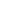RECHERCHER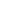PARCOURIR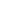LIBRE ACCÈS